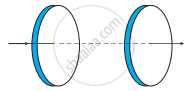# A Capacitor Made of Two Circular Plates Each of Radius 12 cm, and Separated by 5.0 cm. the Capacitor is Being Charged by an External Source - Physics

Numerical

Figure shows a capacitor made of two circular plates each of radius 12 cm, and separated by 5.0 cm. The capacitor is being charged by an external source (not shown in the figure). The charging current is constant and equal to 0.15 A.

(a) Calculate the capacitance and the rate of charge of potential difference between the plates.

(b) Obtain the displacement current across the plates.

(c) Is Kirchhoff’s first rule (junction rule) valid at each plate of the capacitor? Explain.#### Solution

Radius of each circular plate, r = 12 cm = 0.12 m

Distance between the plates, d = 5 cm = 0.05 m

Charging current, I = 0.15 A

Permittivity of free space, ε0 = 8.85 × 10−12 C2 N−1 m−2

(a) Capacitance between the two plates is given by the relation,

C =(ε_0"A")/"d"

Where,

A = Area of each plate = πr2

"C" = (ε_0pi"r"^2)/"d"

= (8.85 xx 10^-12 xx pi xx (0.12)^2)/0.05

= 8.0032 × 10−12 F

= 80.032 pF

Charge on each plate, q = CV

Where,

V = Potential difference across the plates

Differentiation on both sides with respect to time (t) gives:

("dq")/("dt") = "C"("dV")/("dt")

But, ("dq")/("dt") = current (I)

∴ ("dV")/("dt") = "I"/"C"

= (0.15)/(80.032 xx 10^-12)

= 1.87 × 109 V/s

Therefore, the change in the potential difference between the plates is 1.87 ×109 V/s.

(b) The displacement current across the plates is the same as the conduction current. Hence, the displacement current, id is 0.15 A.

(c) Yes

Kirchhoff’s first rule is valid at each plate of the capacitor provided that we take the sum of conduction and displacement for current.

Concept: Displacement Current
Is there an error in this question or solution?

#### APPEARS IN

NCERT Physics Part 1 and 2 Class 12
Chapter 8 Electromagnetic Waves
Exercise | Q 8.1 | Page 285
NCERT Class 12 Physics Textbook
Chapter 8 Electromagnetic Waves
Exercise | Q 1 | Page 286

Share